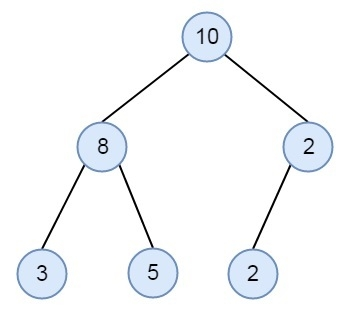# Check for Children Sum Property in a Binary Tree in C++

Suppose we have a binary tree. The binary tree is valid when it meets the following property.

• Each node should contain data value same as the sum of left and right children values. If there are no children at any side, then it will be treated as 0.

Suppose a tree is present like below, which meets the given property.There is no such trick to check this, we have to traverse the tree recursively, if the node and both of its children satisfies the property then return true, otherwise false.

## Example

#include <iostream>
using namespace std;
class node {
public:
int data;
node* left;
node* right;
};
bool isValidBinaryTree(node* nd) {
int left_data = 0, right_data = 0;
if(nd == NULL || (nd->left == NULL && nd->right == NULL))
return 1;
else{
if(nd->left != NULL)
left_data = nd->left->data;
if(nd->right != NULL)
right_data = nd->right->data;
if((nd->data == left_data + right_data)&& isValidBinaryTree(nd->left) && isValidBinaryTree(nd->right))
return true;
else
return false;
}
}
node* getNode(int data) {
node* newNode = new node();
newNode->data = data;
newNode->left = NULL;
newNode->right = NULL;
return newNode;
}
int main() {
node *root = getNode(10);
root->left = getNode(8);
root->right = getNode(2);
root->left->left = getNode(3);
root->left->right = getNode(5);
root->right->right = getNode(2);
if(isValidBinaryTree(root))
cout << "The tree satisfies the children sum property ";
else
cout << "The tree does not satisfy the children sum property ";
}

## Output

The tree satisfies the children sum property